A# number base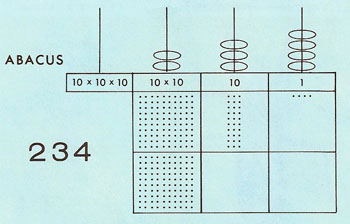Fig 1. The number 234 written in decimal.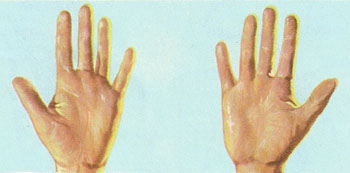Fig 2. Human hands.Fig 3. Martian hands.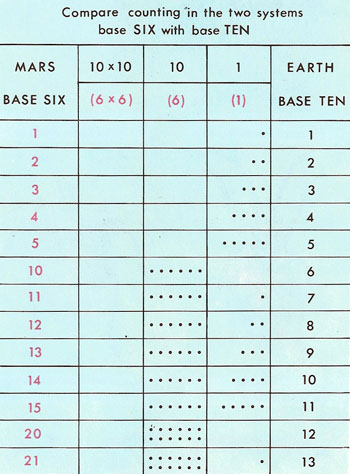Fig 4. Counting in bases six and ten.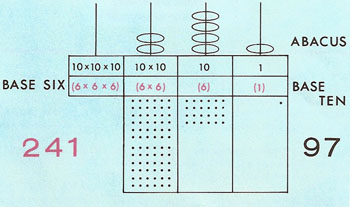Fig 5. The number 241 in the base six system.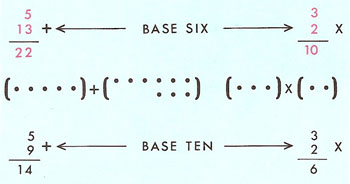Fig 6. The number 241 in the base six system.

A number base is the number upon which a number system is based; this is also the number of different characters or figures needed by the number system.

The base, or radix, of our familiar decimal system is 10. Thus, there are ten symbols, 0, 1, 2, 3, 4, 5, 6, 7, 8, and 9, and a decimal number is written right to left in terms of units, tens, hundreds, and so on. Each move to the left represents a jump by a power of 10. The decimal number 375, for example, equals (3 × 102) + (7 × 10) + (5 × 1). This can easily be written to another base. Decimal 37510 becomes in octal (base 8) 5678 = (5 × 82) + (6 × 8) + (7 × 1), or in binary (base 2) 1011110012 = (1 × 28) + (0 × 27) + (1 × 26) + (1 × 25) + (1 × 24) + (1 × 23) + (0 × 22) + (0 × 2) + (1 × 1).

## A Martian number base?

Imagine trying to explain to an intelligent being on another planet, say Mars, how our number system works (Fig 1). We should have to explain that in a number such as 234 the right-hand number refers to the units, the next numbers to the numbers of 10's, and the next to the number of 100's (10 × 10). Our number system is based on tens because we happen to have ten fingers and thumbs on our hands (Figure 2).

"We number the fingers in the same way as you do. The last one is written 10, but because we have only six fingers we have only six digits in all (0, 1, 2, 3, 4, 5)." So the Martians, if they existed, would use a base of six.

Figure 4 shows how the same numbers would be written by the Martian,using his base of six, and by us using our base of ten. The last number of both bases is written 10.

Now let's look at a three-figure number written using a base of six, for example 241 (Fig 5). The right-hand number is 1, as in our decimal system. In the decimal system the middle number would mean 4 × 10; in the base six system it equals 4 × 6. In the same way, where the left-hand number would be 2(10 × 10) in the decimal system, in the base six system it is 2(6 × 6). So 241 in the base six system corresponds to 2(6 × 6) + 4 × 6 + 1 in our system, which adds up to 97.

In the base six system the "bonds" of addition and multiplication are different from ours, so calculations look rather odd (Fig 6).